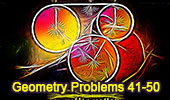# Ten Geometry Problems 41-50. Level: High School, College# Ten Geometry Problems 41 - 50Sangaku Problem 41.
"Mickey Mouse." Three circles, tangents, radii.

Problem 42.
Angles, equal segments, and triangles, 30-60 degrees.

Problem 43.
Angles equal segments, and triangles, 30-60 degrees.

Art and typography of problem 43.

Problem 44.
Angles and triangles, 30-60 degrees.

Problem 45.
Angles and triangles, 30-60 degrees.

Problem 46.
Triangle, median, and angles, 30 degrees.

Problem 47.
Triangle, median, and angles, 15, 30 degrees.

Problem 48.
Angles and triangles, 30 degrees.

Problem 49
Right triangle, cevian, perpendicular, and angles.

Problem 50.
Triangle with Equilateral triangles. 17 Propositions
.

 Home | Sitemap | Search | Geometry | Problems | Visual Index | 10 Problems | Email | Post a comment | By Antonio Gutierrez Last updated: Mar 7, 2018